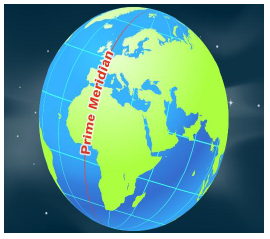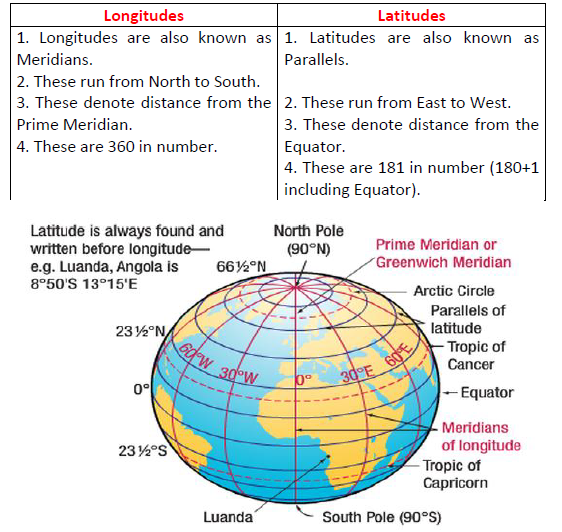# CBSE Class 5 Social Science The Globe The Model of The Earth Worksheet

Download printable Social Science Class 5 Worksheets in pdf format, CBSE Class 5 Social Science The Globe The Model of The Earth Worksheet has been prepared as per the latest syllabus and exam pattern issued by CBSE, NCERT and KVS. Also download free pdf Social Science Class 5 Assignments and practice them daily to get better marks in tests and exams for Grade 5. Free chapter wise worksheets with answers have been designed by Standard 5 teachers as per latest examination pattern

## Worksheet for Class 5 Social Science The Globe The Model of The Earth

Class 5 Social Science students should refer to the following printable worksheet in Pdf for The Globe The Model of The Earth in Grade 5. This test paper with questions and solutions for Standard 5 Social Science will be very useful for tests and exams and help you to score better marks

### The Globe The Model of The Earth Class 5 Social Science Worksheet Pdf

THE GLOBE -THE MODEL OF THE EARTH

Answer the following questions in brief:

Q.1. Why Globe is called as model of the Earth?
Ans. Because Globe shows accurate sizes and shapes of all the physical features of the earth, such as oceans and continents.

Q.2. What is the name of the ending of screw of Globe?Ans. The upper screw is called North Pole and lower one is called South Pole.

Q.3. What is the name given to the Semi-circles that connect both the poles?
Ans. Longitudes.

Q.4. What is Hemisphere, name the line that cuts the Globe or Earth into the Hemispheres?
Ans. Equator is the imaginary line that divides the Earth in two equal halves. Each half is known as hemisphere.

Q.5. What is the Prime Meridian, from where it passes?
Ans. Counting of meridians begin from Prime Meridian. Its value is 00 Longitude. It passes through Greenwich in England.Q.6. Why Longitudes are called Meridians of Longitude. In how many Meridians we divide the Earth or Globe?
Ans. Meridians are great circles which are not parallel. Longitudes are also not parallel. That’s why Longitudes are called Meridians of Longitude.

Q.7. Which is the largest circle of Globe, name it?
Ans. The circle of Equator.

Q.8. What is the difference between Parallel of Longitude and Meridian of Latitude?
Ans.Q.9. What is the importance of Longitude?
Ans. 1. With the help of Longitudes, we are able to know the East- West distance of a place.
2. We can calculate the local time of a place.

Q.10. Which lines help us to divide earth into Heat zones? Give reasons why there are Heat Zones?
Ans. Latitudes divide earth into Heat zones.
Earth is spherical in shape. The central part of the earth receives maximum heat of the Sun and as we go towards the poles, the heat reduces. That’s why there are heat zones.

Fill in the Blanks:

1. Tropic of Cancer is depicted by 23½0 N parallels of Latitude.

2. Tropic of Capricorn is depicted by 23½0 S parallels of Latitude.

3. Latitudes and Longitudes make a grid on a Globe.

4. The distance between any two parallels is always equal.

5. The Prime Meridian is also known Zero Meridian.

6. In India, the Longitude of 82½0 E is considered the Standard Meridian.

7. The time difference between Greenwich Mean Time and Indian Standard Time is 5 hours and 30 minutes.

Say whether the following statements are True or False:

1. Each parallel of Latitude is a semi-circle. False

2. Temperature increases as we go away from the equator. False

3. The Torrid Zone lies between the Tropic of Cancer and Tropic of Capricorn. True

4. The Earth rotates from the West to East direction. True

More questions-

I NAME THE FOLLOWING:

1. Imaginary lines that are at equal distance from each other __________

2. The imaginary line around which the Earth spins. _______

3. It divides the earth into the Northern and Southern Hemisphere._________

4. The latitudes and longitudes intersect each other at right angles and form a series of squares _______

5. It measures the distance of a place north or south of the Equator- _____________

6. It measures the distance of a place east or west of the Prime Meridian. _______________

7. The important tools used by Geographers to represent the Earth on asmaller scale- __________, __________

8. The latitude of 66½ 0 S - _____________________

9. The latitude of 23½ 0 N - _____________________

II FILL IN THE BLANKS:

1. The __________ is a small model of the Earth.

2. The two endpoints of the axis of rotation are called the _______.

3. The _____________ is an imaginary line that circles the Earth midway between the poles.

4. The Equator divides the Earth into two equal halves called the ________________

5. The axis is tilted at an angle of _________

6. Latitudes and Longitudes are marked in ____________ and ___________ respectively.

7. There are ______ degrees of latitude in all.

8. A _______ helps us to locate places on maps and globes.

9. The _______________ decreases in length as you move from the equator to the poles.

10.The ___________________ run from the North Pole to the South Pole.

11.Longitude 0 0 is called the _____________________________ or the _________________________

12.The 1800 East and 1800 West longitudes meet and form a single line called the _________________________________

III IDENTIFY THE PICTURE AND FILL IN THE BLANKS:a. This is a picture of the ____________.

b. The direction of rotation is from ________ to ___________.

c. The measurement of the North Pole is ______.

d. These lines are at an equal distance from each other. __________

IV ANSWER THE FOLLOWING QUESTIONS IN 2 POINTS:

1 State the uses of a globe.

2 What do you know about the 00 Meridian?

3 Why does the earth appear flat to us?

V ANSWER THE FOLLOWING QUESTION IN 3 POINTS:

4 Write a short note on Eartha.

VII ANSWER THE FOLLOWING QUESTIONS IN FOUR POINTS:

5 Explain the features of Latitudes.

6 Describe the features of Longitudes.